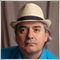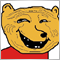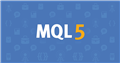# 2 Different indicators in the same window [Code Help]894

Hello all!

I'm trying to make a custom indicator that's able to plot RSI and OBV on the same window so I don't have to load those indicators again and again but I'm having trouble to normalize the value of OBV to fit the range of RSI or ignoring the range of the RSI and just show it relative to its position on the window just as if I throw 2 indicators on the same window. Here's the code I'm using:

```int start()
{
//---
// More vars here too...
int start = 0;
int limit;
int counted_bars = IndicatorCounted();

// check for possible errors
if(counted_bars < 0)
return(-1);

// Only check these
limit = Bars - 1 - counted_bars;
if(counted_bars==0) limit-=1+1;

// Check the signal foreach bar
for(int i = limit; i >= start; i--)
{
double RSI_Val = iRSI(Symbol(),Period(),RSI_Period,PRICE_CLOSE,i);
double OBV_Val = iOBV(Symbol(),Period(),ENUM_APPLIED_PRICE(OnBalanceVolumeApplyTo),i);

if(i > 0)
{
if(OBV_Val > OBV_Max)
{
OBV_Max = OBV_Val;
}
else if(OBV_Val < OBV_Min)
{
OBV_Min = OBV_Val;
}
}

ExtMapBuffer0[i] = RSI_Val;
ExtMapBuffer1[i] = (100-0)*((OBV_Val - OBV_Min)/(OBV_Max - OBV_Min))+0;

if(Time[i] == Time)
{
}
}

//--- return value of prev_calculated for next call
return(0);
}```

I know its not the most efficient or readable, it's my first time working on indicators, I mostly work with EA. The logic I was using to normalize it its the one on this thread: https://stats.stackexchange.com/questions/178626/how-to-normalize-data-between-1-and-1

Is this possible or there's a way to get the desired result (Upper one is the one generated with the code and the bottom one my desired result):How to normalize data between -1 and 1?
• stats.stackexchange.com
I have seen the min-max normalization formula but that normalizes values between 0 and 1. How would I normalize my data between -1 and 1? I have both negative and positive values in my data matrix.4896

Fernando Jose Velasco Borea: I'm trying to make a custom indicator that's able to plot RSI and OBV on the same window so I don't have to load those indicators again and again but I'm having trouble to normalize the value of OBV to fit the range of RSI or ignoring the range of the RSI and just show it relative to its position on the window just as if I throw 2 indicators on the same window. Here's the code I'm using:

I know its not the most efficient or readable, it's my first time working on indicators, I mostly work with EA. The logic I was using to normalize it its the one on this thread: https://stats.stackexchange.com/questions/178626/how-to-normalize-data-between-1-and-1

Is this possible or there's a way to get the desired result (Upper one is the one generated with the code and the bottom one my desired result):

In an indicator, all the buffers share the same units, so you can't have buffers with different units. You could scale them, but then their values would not make sense, neither on the graph nor in the Data Window.

If you really want them to share the same sub-window, then just drop the two individual indicators onto the same sub-window and then save it as a Template, which you can then later load in other charts in order to load them both together with colours and parameters set and all.894

Fernando Carreiro:

In an indicator, all the buffers share the same units, so you can't have buffers with different units. You could scale them, but then their values would not make sense, neither on the graph nor in the Data Window.

If you really want them to share the same sub-window, then just drop the two individual indicators onto the same sub-window and then save it as a Template, which you can then later load in other charts in order to load them both together with colours and parameters set and all.

Yep, tried that but the problem is that if I have some charting it will be lost when I load the template and otherwise I will have to save an individual template for each pair:/858

Fernando Jose Velasco Borea:

Hello all!

I'm trying to make a custom indicator that's able to plot RSI and OBV on the same window so I don't have to load those indicators again and again but I'm having trouble to normalize the value of OBV to fit the range of RSI or ignoring the range of the RSI and just show it relative to its position on the window just as if I throw 2 indicators on the same window. Here's the code I'm using:

I know its not the most efficient or readable, it's my first time working on indicators, I mostly work with EA. The logic I was using to normalize it its the one on this thread: https://stats.stackexchange.com/questions/178626/how-to-normalize-data-between-1-and-1

Is this possible or there's a way to get the desired result (Upper one is the one generated with the code and the bottom one my desired result):